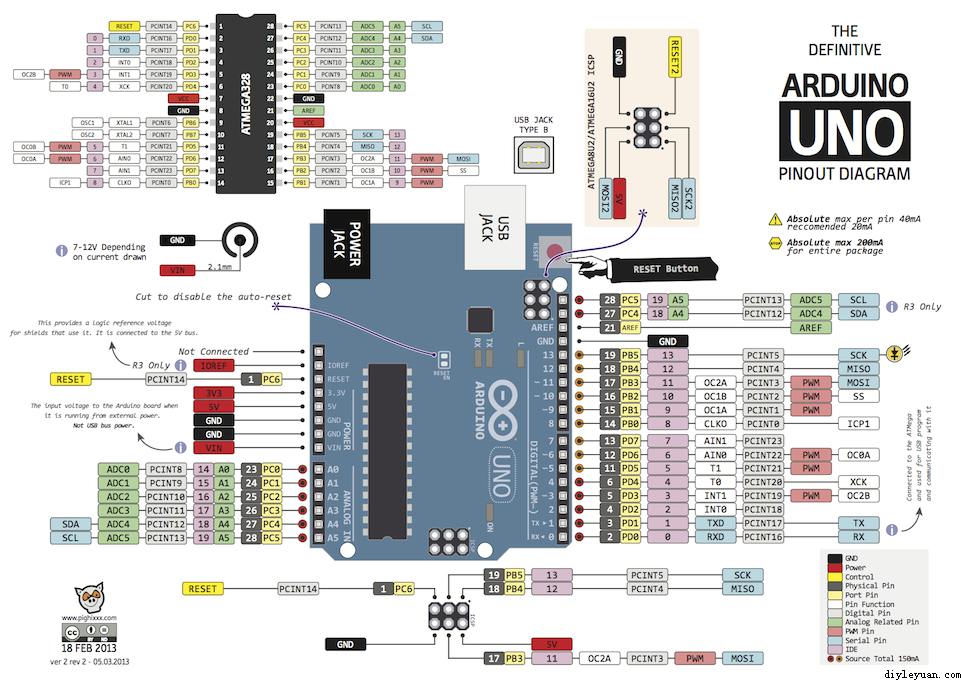```int  n=255;
const int PWMPin=6;

void setup()
{
Serial.begin(9600);
pinMode(PWMPin,OUTPUT);      //该端口需要选择有#号标识的数字口
}

void loop()
{
char  c;

while (Serial.available() > 0)
{
if (']'==c)
{
n=n+5;
}
if ('['==c)
{
n=n-5;
}
if (n>255) {n=0;}
if (n<0) {n=255;}
analogWrite(PWMPin,n);
Serial.println(n);

}
}```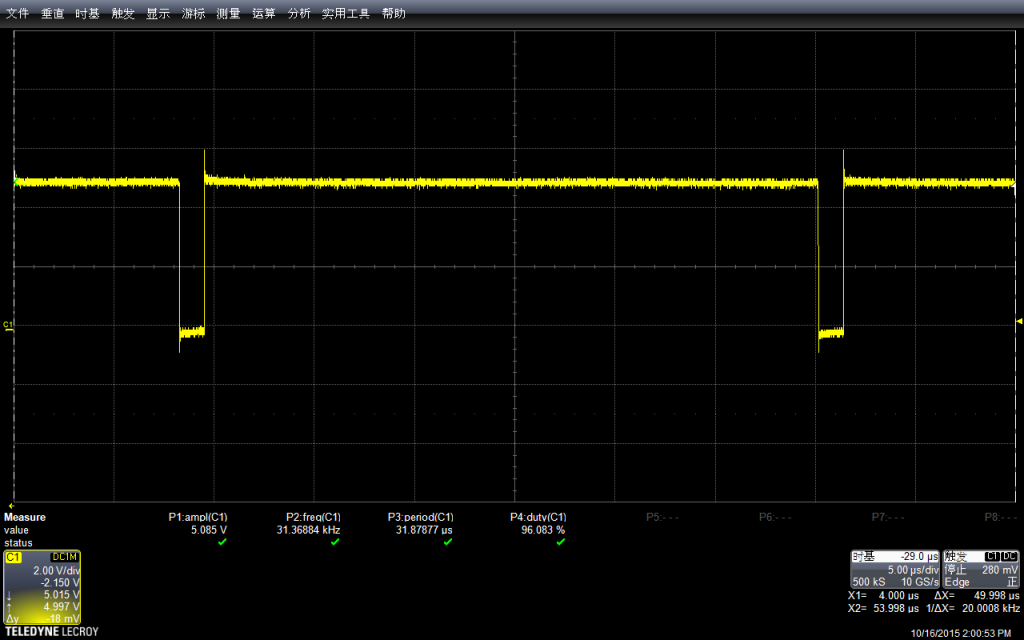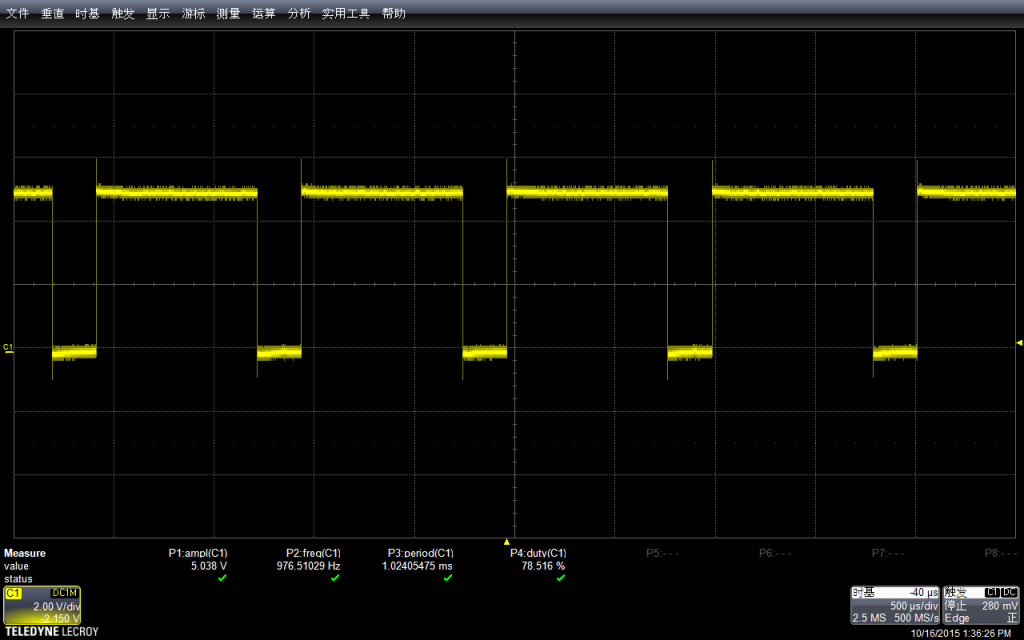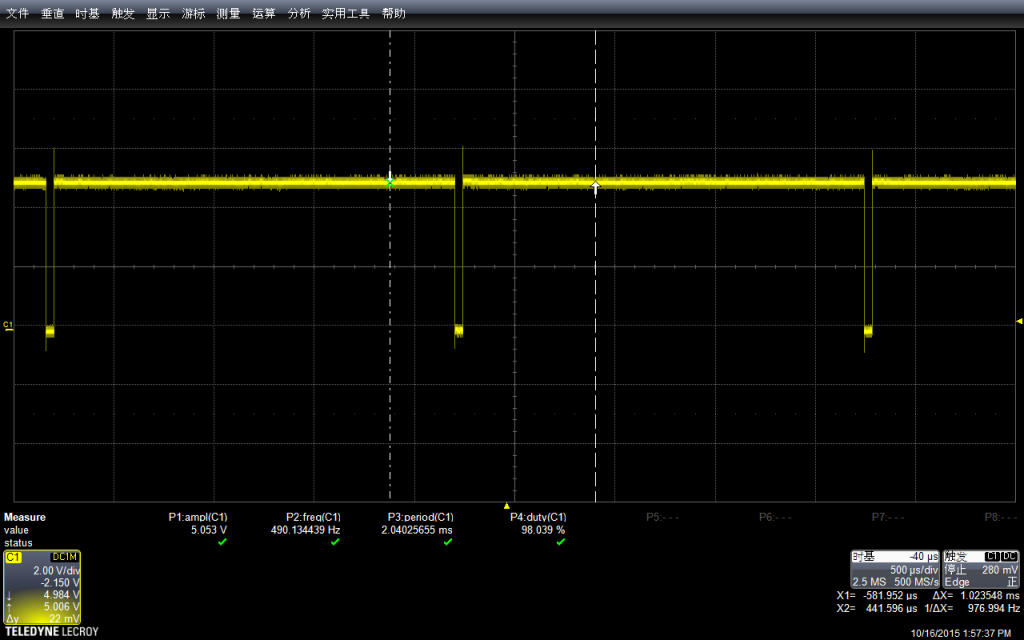```int  n=255;
const int PWMPin=9;

void setup()
{
Serial.begin(9600);
TCCR1B = TCCR1B & B11111000 | B00000001;    // set timer 1 divisor to 1 for PWM frequency of 31372.55 Hz
pinMode(PWMPin,OUTPUT);      //该端口需要选择有#号标识的数字口
}

void loop()
{
char  c;

while (Serial.available() > 0)
{
if (']'==c)
{
n=n+5;
}
if ('['==c)
{
n=n-5;
}
if (n>255) {n=0;}
if (n<0) {n=255;}
analogWrite(PWMPin,n);
Serial.println(n);

}
}```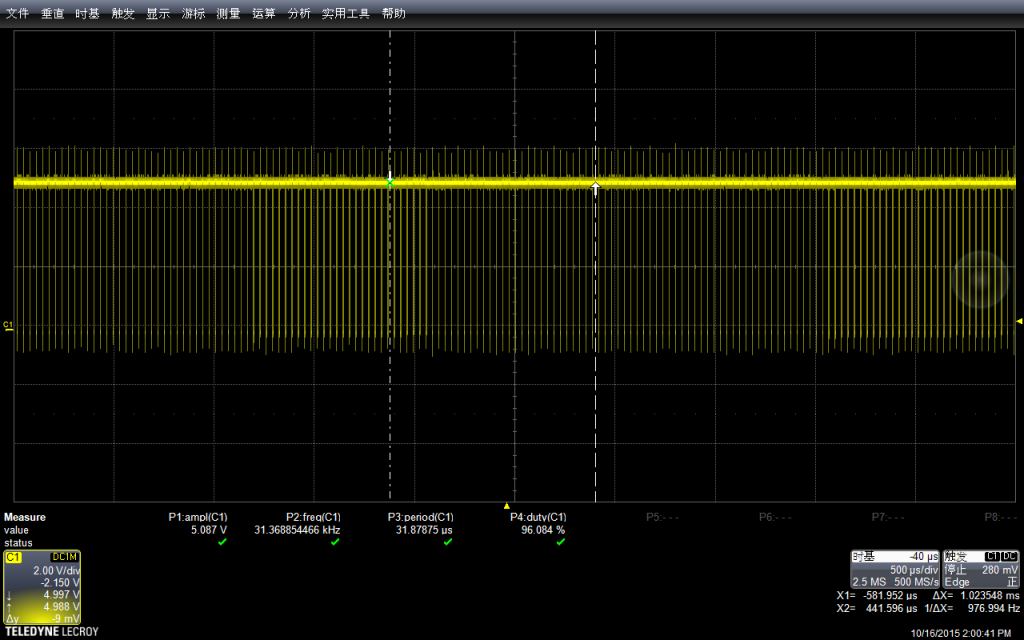1. http://www.diyleyuan.com/index.php?m=content&c=index&a=show&catid=29&id=616
2. https://arduino-info.wikispaces.com/Arduino-PWM-Frequency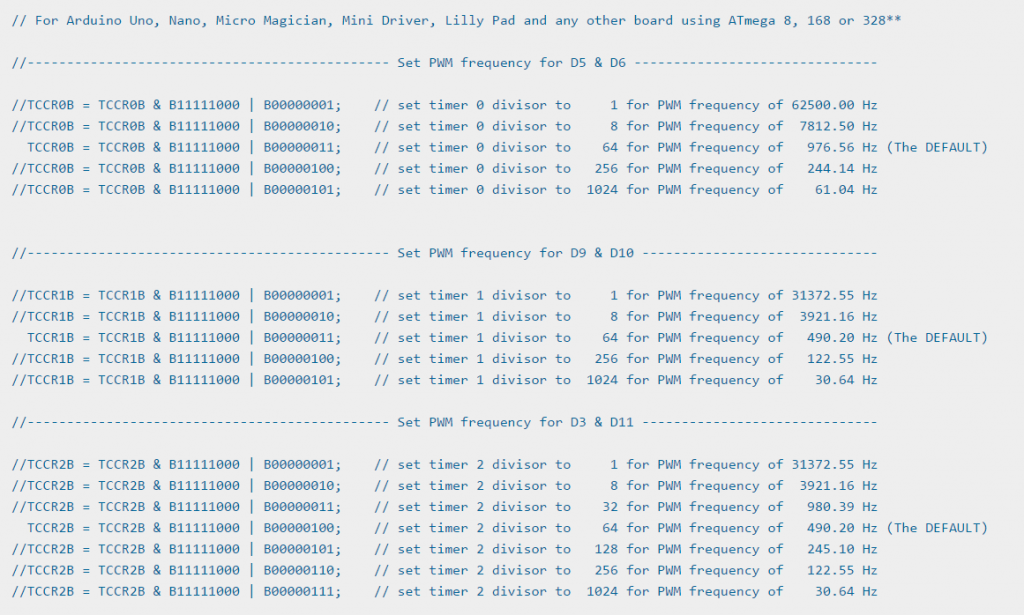3. http://playground.arduino.cc/Code/PwmFrequency 关于调整频率官方的介绍
4. https://www.arduino.cc/en/Tutorial/SecretsOfArduinoPWM Secrets of Arduino PWM
http://www.diy-robots.com/?p=852 上面文章的翻译
http://www.diy-robots.com/?p=814 上面文章的翻译